#### Sampling rates

Signal processing formulations exist for both continuous-time, which makes nice formulations and mathematical proofs, and discrete-time, which has an uglier appearance, but corresponds directly to the way computers process signals. Because of its practical value, we will focus on the discrete-time case.

Start with a signal as a function of time, with values represented as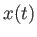. Using digital processing, it will be sampled at regular time intervals. Let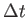be the sampling interval. The sampling rate or (sampling frequency) roughly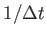Hz. For example, with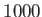Hz sampling frequency,is one millisecond. According to the Nyquist-Shannon sampling theorem, the sampling rate should be at least two times the highest frequency component in the signal. Since the highest frequency component for audio is 20,000 Hz, this suggests that the sampling rate should be at least 40,000 Hz. By no coincidence, the sampling rate of CDs and DVDs are 44,100 Hz and 48,000 Hz, respectively.

By sampling the signal, an array of values is produced.11.1 AtHz, the array would contain a thousand values for every second. Using an index variable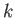, we can refer to theth sample as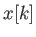, which corresponds to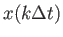. Arbitrarily, the first sample is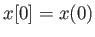.

Steven M LaValle 2020-11-11